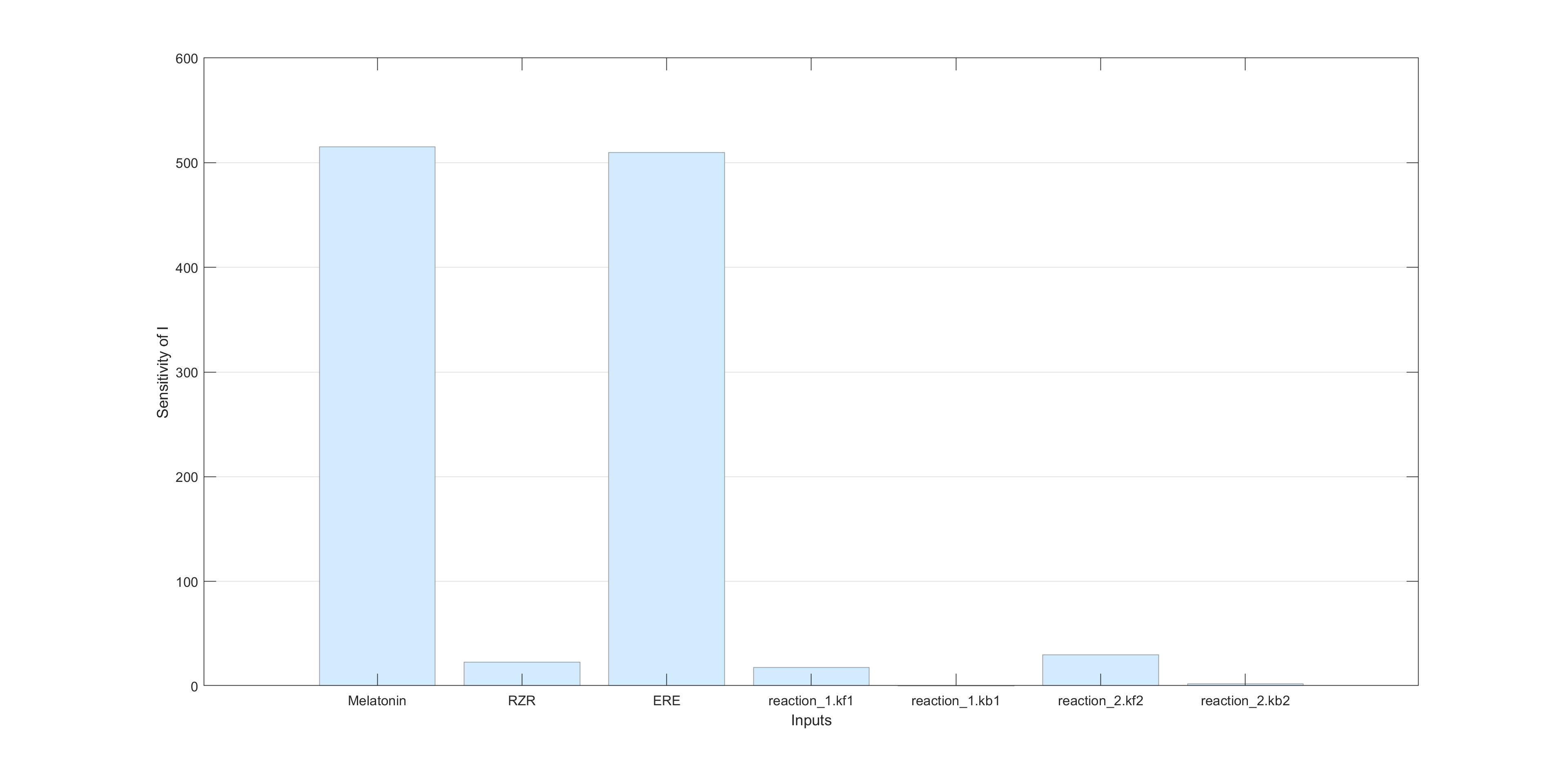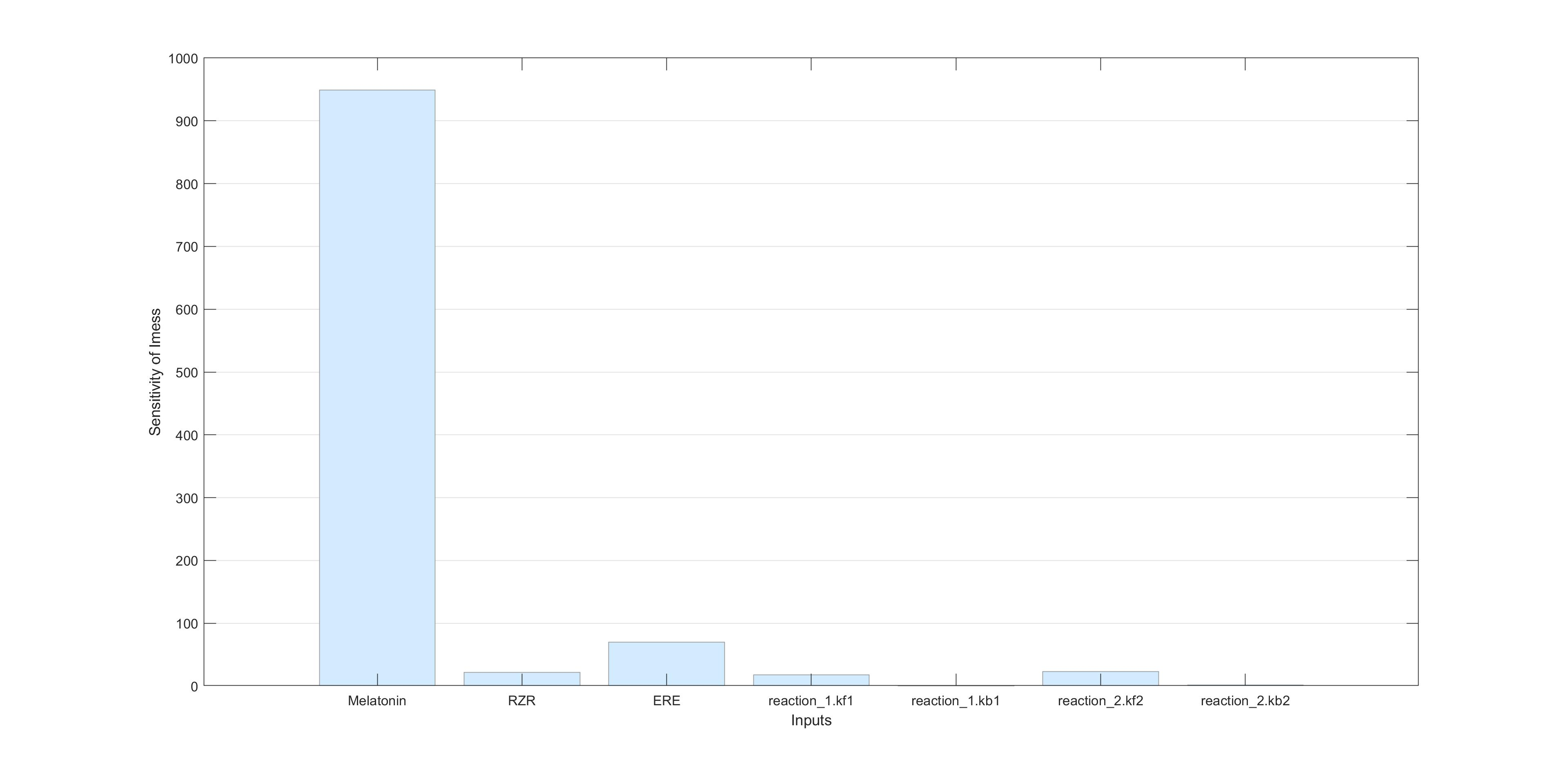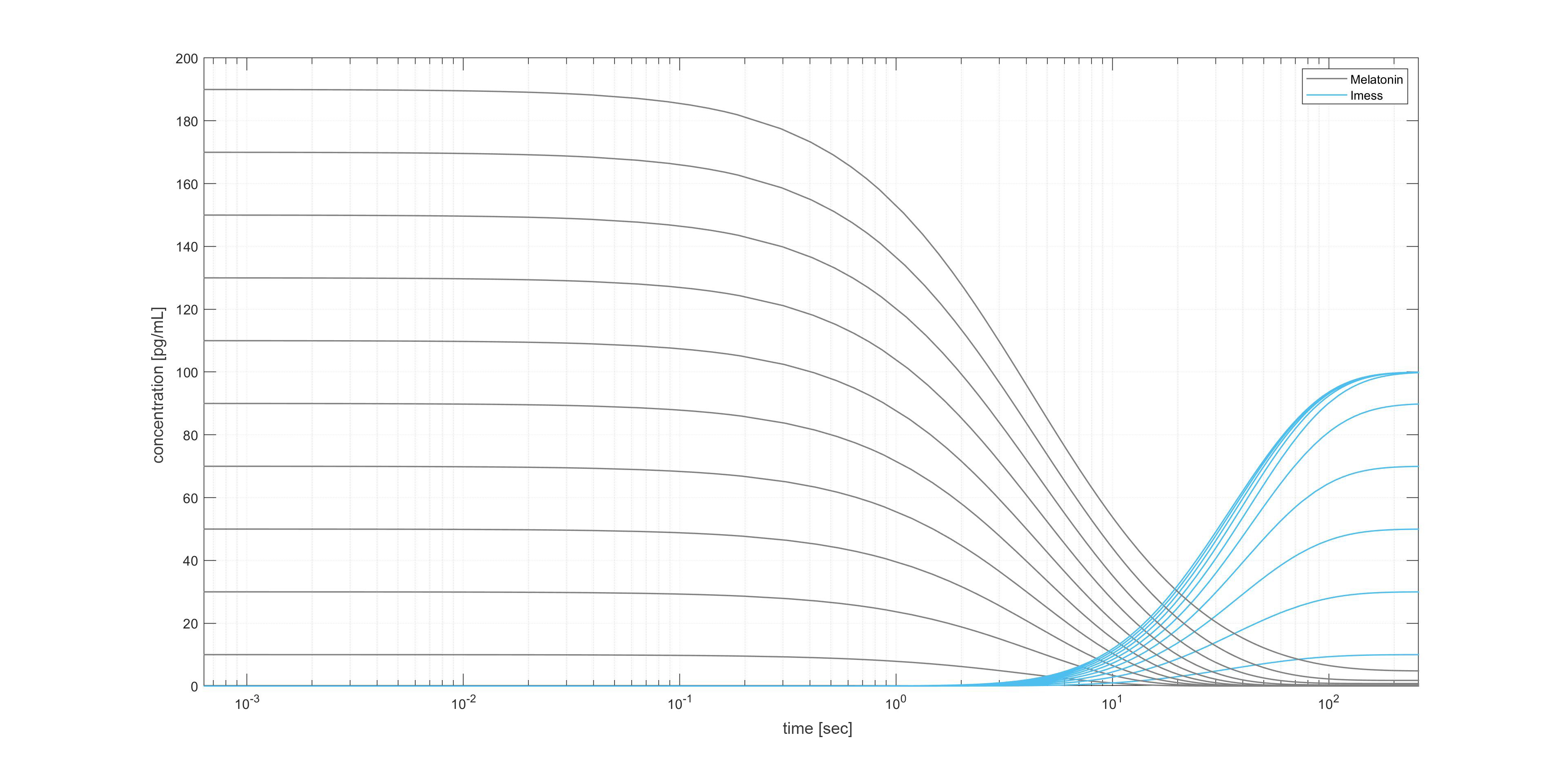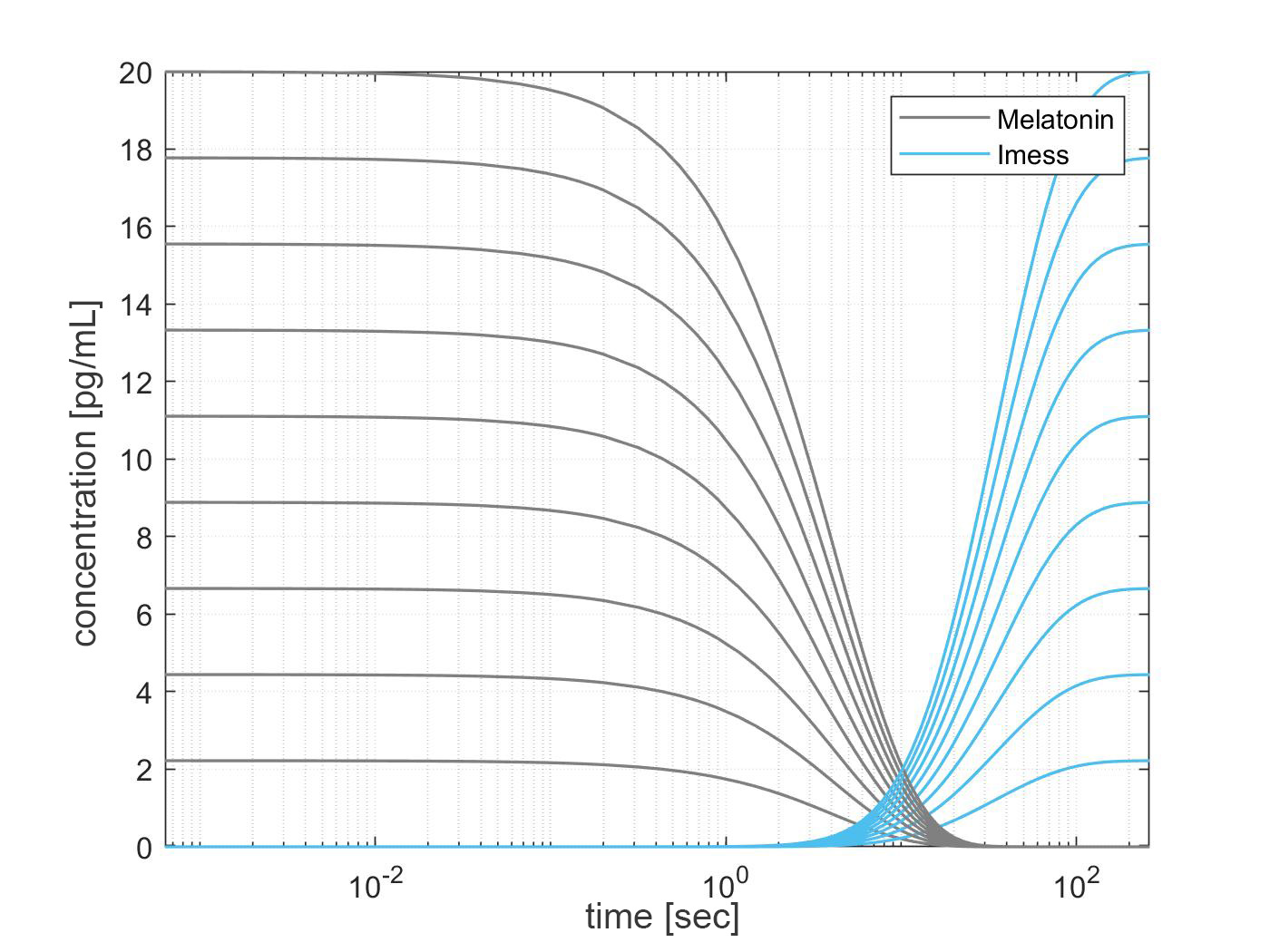# Modelling

## Combined model of the biological project part and the hardware

The goal of modelling in our project is to understand how the reactions take place in our systems. Furthermore, it is possible to find out which parameters are essential for the sensitivity and more important you can predict a calibration line, where the Melatonin-sensor is working. All models were simplified and are not representing the equivalent equations or represent the reality.

### Model Structure

Before starting to build the model it is necessary to have knowledge about the structure of a model and more important the different types of enzyme kinetics. In this small chapter the two types of kinetics, which were used in our model, are explained.

##### Enzyme kinetics

Enzyme kinetics is part of biophysical chemistry. It describes how fast reactions are taking place and is often used in biology or medicine. One main goal is to understand the process and deduce information, e.g. about the sensitivity of parameters.
Leonor Michaelis and Maud Menten could confirm Victor Henri’s theory with experiments. The key idea behind the theory is to separate the enzyme reaction into two parts. The first one is the binding of the substrate S with the enzyme E and the following is the forming of the product P and the enzyme E.

##### Hill-Kinetics

Another concept on how to describe the enzyme kinetic is the Hill kinetic. It is an important model for regulation processes, which take place in reactions. The reaction rate can be expressed in this way:

In figure 2 a matlab plot of a hill kinetic is shown. After a specific amount of a substrate the product is produced. This is important for the description of gene expression.

### Building the model

To build the model we used the Matlab tool SimBiology. The benefit of the tool is that you can start building the model without knowledge in programming a code. The tool is based on a visual programming language, where you can change the kinetics and the parameters. It is a strong tool to build a complex model and thereby fits perfect for our goals.

##### Biological model

The biological model is based on Michaelis-Menten kinetic and Hill kinetic. In figure 3 the model is shown.

The model is divided into 5 reactions. In the first step there is a reaction between Melatonin and the nuclear receptor retinoid Z receptor (RZR), which is added in the process and can be seen as constant. The reaction is reversible and is described with a normal enzyme kinetic. After that, the RZR-melatonin complex binds on a specific estrogen response element (ERE), where the luciferase gene is expressed. It is expressed by the reactions 2 and 3. The gene expression is described by the hill kinetic. After a specific time, the luciferase is expressed. In the next step, luciferase reacts with luciferin to luciferase, photons (light) and oxy-luciferin. This reaction is described by a mass kinetic. The luciferase in the system also degenerates and can not be used anymore for the reaction.
One possible simulation of the concentration is shown in figure 4.

##### Hardware model

With the knowledge of the biological model the hardware model is built. There are a few changes between the biological part and the hardware part. The first two reactions are the same. Melatonin reacts with RZR to a complex, which binds to the ERE, but in this case, there is no gene expression. We decided to measure the light intensity with localized surface plasmon resonance (LSPR) instead of using luciferase. The most important reason for the change is that it enables us to measure outside a lab.

The reaction 3, which describes the shift of light wavelength, cannot be described by a normal kinetic. It is a complex reaction, which should be modelled in a different way. Drude model electronic conduction and the Mie-Gans theory are applied to describe how the LSPR wavelength changes during cation exchange, taking into account the morphology evolution and changes to the local permittivity (Analytical modelling of localized surface plasmon resonance in heterostructure copper sulfide nanocrystals).

For our case this method is way too complex to describe. So, we tried to express the LSPR reaction with an imaginary approximation function. With that, the reaction can be described roughly for a specific range of melatonin. In the last step, the wavelength of the light with no binding is compared with the light with the bounded complex. In near future, this reaction should be expressed by a specific function or with a complex model.
In figure 7 a possible simulation for the hardware device is shown.

### Sensitivity analysis

One important benefit of SimBiology is that you can make a sensitivity analysis in an easy way. For both models, the sensitivity analysis is made until reaction 2. As input parameters melatonin, RZR, ERE and the reaction rates were chosen. The bounded melatonin-RZR complex on the ERE is chosen as the output parameter. In the biological model, the production of luciferase and in the hardware model the intensity of the light is a function of the bounded melatonin-RZR complex on the ERE. The only difference is that the amount of ERE changes in both models. In this case, it is possible to make just one analysis to get information about the sensitivity of both models.

Figure 8 shows the different weightings of the parameters. Depending on the initial concentration the weighting changes. As RZR is present in excess, it has not a high impact on the sensitivity of the light intensity. Ergo, there is no reason to change this factor. Other parameters like melatonin have a higher impact on the system. If the concentration of melatonin is lowered, even by 10 percent, melatonin is the most sensitive parameter in the system. If the concentration is increased, it is the opposite. The problem with melatonin is that it is not a factor which could be manipulated as an input parameter because we want to measure it. Changing the reaction rates influences the sensitivity as well but is harder to realize. By lowering one of the reaction rate factors kf or kb drastically by the factor 10 the sensitivity of those parameters increases. The lower the reaction rate constant the higher is the influence in the system. The easiest factor which can be regulated in the system is the amount of ERE. The reaction rates can be manipulated by biological engineering.Figure 8 a): Sensitivy analysis: starting weightings of the parametersFigure 8 b): Sensitivy analysis: example of changes in the sensitivity by changing the concentration of melatonin

### Hardware calibration

The last part of the analysis of the model is creating a calibration line. It is important to know in which concentrations the device is working. In this case, the model simulates different melatonin concentrations and predicts the measured light intensity. The resolution with low melatonin concentration is relatively high. It is possible to measure lower melatonin concentrations, but they might be not exact and could have a deviation, depending on the resolution of the spectrometer.Figure 9 a): Matlab plot for different melatonin concentrations to test the range of the sensorFigure 9 b): one section of the plot zoomed in

Melatonin concentrations higher than 190 pg/ml are not measurable anymore. It is important to adjust the device for a specific range where all possible concentrations are measurable. For the sensitive analysis it is important to know which parameter has the most influence.